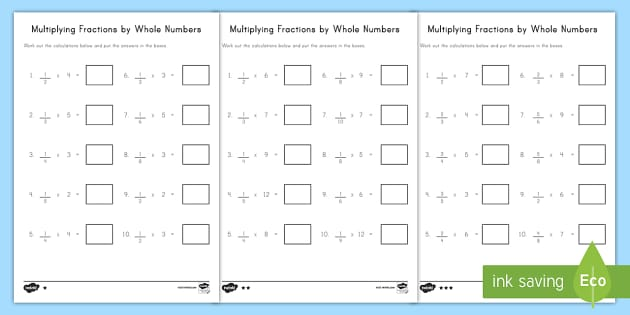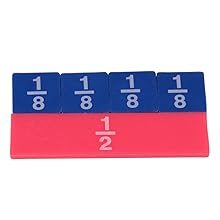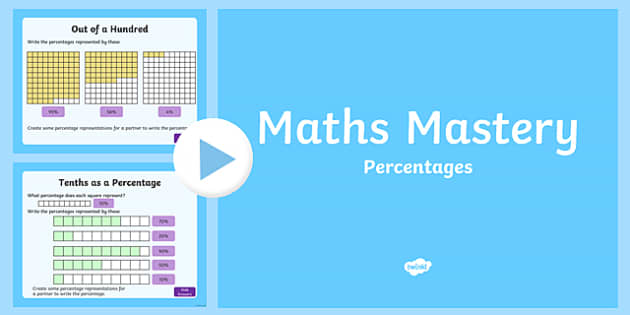# Fractions 2

A fraction like 34 says we have 3 out of the 4 parts the whole is divided into.

### To add fractions there are Three Simple Steps:

• Step 1: Make sure the bottom numbers (the denominators) are the same
• Step 2: Add the top numbers (the numerators), put that answer over the denominator
• Step 3: Simplify the fraction (if needed)

## Example:

Step 1. The bottom numbers (the denominators) are already the same. Go straight to step 2.

Step 2. Add the top numbers and put the answer over the same denominator:

14 + 14 = 1 + 14 = 24Step 3. Simplify the fraction:14 + 14 = 24 = 12

.. and do you see how 24 is simpler as 12 ? (see Equivalent Fractions.)

## Example:

13 + 16

### Fractions Calculator - RapidTables.com

Step 1: The bottom numbers are different. See how the slices are different sizes?

 13 + 16 = ?

We need to make them the same before we can continue, because we can't add them like that.

The number '6' is twice as big as '3', so to make the bottom numbers the same we can multiply the top and bottom of the first fraction by 2, like this:

 × 2
 × 2

Important: you multiply both top and bottom by the same amount,
to keep the value of the fraction the same

Now the fractions have the same bottom number ('6'), and our question looks like this:

 26 + 16

The bottom numbers are now the same, so we can go to step 2.

Step 2: Add the top numbers and put them over the same denominator:

26 + 16 = 2 + 16 = 36

In picture form it looks like this:

 26 + 16 = 36

Step 3: Simplify the fraction:

36 = 12

In picture form the whole answer looks like this:

 26 + 16 = 36 = 12

### With Pen and Paper

And here is how to do it with a pen and paper (press the play button):

The bottom numbers must be the same!

'Change the bottom using multiply or divide,
But the same to the top must be applied,

'And don't forget to simplify,
Before its time to say good bye'

## Example:

13 + 15

Again, the bottom numbers are different (the slices are different sizes)!

 13 + 15 = ?

But let us try dividing them into smaller sizes that will each be the same:

 515 + 315

The first fraction: by multiplying the top and bottom by 5 we ended up with 515 :

 × 5
 13 = 515
 × 5

The second fraction: by multiplying the top and bottom by 3 we ended up with 315 :

 × 3
 × 3

The bottom numbers are now the same, so we can go ahead and add the top numbers:

 515 + 315 = 815

The result is already as simple as it can be, so that is the answer: 815

13 + 15 = 815

## Making the Denominators the Same

In the previous example how did we know to cut them into 1/15ths to make the denominators the same? We simply multiplied the two denominators together (3 × 5 = 15).

Read about the two main ways to make the denominators the same here:

• Common Denominator Method, or the

They both work, use which one you prefer!

### Example: Cupcakes

You want to make and sell cupcakes:• A friend can supply the ingredients, if you give them 1/3 of sales
• And a market stall costs 1/4 of sales

How much is that altogether?

We need to add 1/3 and 1/4

 1 + 1 = ? 3 4 ?

First make the bottom numbers (the denominators) the same.

Multiply top and bottom of 1/3 by 4:

 1 × 4 + 1 = ? 3 × 4 4 ?

And multiply top and bottom of 1/4 by 3:

 1 × 4 + 1 × 3 = ? 3 × 4 4 × 3 ?

Now do the calculations:

 4 + 3 = 4+3 = 7 12 12 12 12

Answer: 712 of sales go in ingredients and market costs.

Do you want to play fun and interactive math fraction games? The list of games on this webpage is designed to reinforce basic skills, while making learning a breeze.

The math games on this page are suitable for elementary and middle school students.

Factors Millionaire Game
Before adding and subtracting fractions, students must understand basic facts about factors and divisibility. This fun game can be used to reinforce these important math concepts.

Factors and Multiples - Jeopardy Game
In this interactive jeopardy game, students will practice finding the greatest common factor (GCF) and the least common multiple (LCM) of two or more numbers.

Soccer Math Game - Simplifying Fractions
In this fun soccer game, students will practice reducing fractions to the simplest form.

Baseball Math Game - Simplifying Fractions
This is a fun baseball math game about game about simplifying fractions. Students will have to first hit a homerun to be able to see and answer a math problem.

Adding and Subtracting Fractions Board Game
Have fun adding and subtracting fractions by playing this interactive board game against another player, or in two teams. This game is an excellent review activity that promotes student engagement.

Equivalent Fractions Game
In this concentration game, students click on two cards to match equivalent fractions. If there is a match, the problems remain on the page; if not, the cards are turned over.

### Fractions - Practice With Math Games

Football Math Game - Adding Fractions
In this game, students will practice adding and subtracting fractions with common and different denominators.

Changing Fractions and Decimals to Percents
Young students can use this fun millionaire game to practice changing decimals and fractions to percents.

Fractions, Decimals, Percents
In this jeopardy game, students will convert fractions to decimals and percents and vice-versa.

### 2nd Grade Fractions - Worksheets, Lessons, And Printables

Multiplying Fractions Game
In this fun and interactive soccer game, students will test their ability to multiply different fractions.

### Fractions 2 - Video Results

Multiplying Fractions Millionaire Game
How many points can you earn? Practice multiplying fractions by playing this fun game alone, with a partner, or in two teams.

Soccer Math - Dividing Fractions Game
Do you know how to divide fractions? Show your friends how many points you can score when playing this fun game.

Math Basketball - Dividing Fractions Game
Dividing fractions does not have to be boring anymore. Play this fun and interactive game and score as many points as possible.

Fractions Jeopardy Game
This online jeopardy game is is a fun way to review the four operations with fractions: addition, subtraction, multiplication, and division.

### More Fractions 2 Images

Changing Fractions to Percents
In this concentration game, students will match different fractions with the equivalent percents.

### Cached

This Fraction Jeopardy Game has two rounds and three categories: fractions, decimals, and percents. The points are doubled in round two. Have fun earning points!

### More Fractions 2 Videos

Return from Math Fraction Games to Math Play homepage.